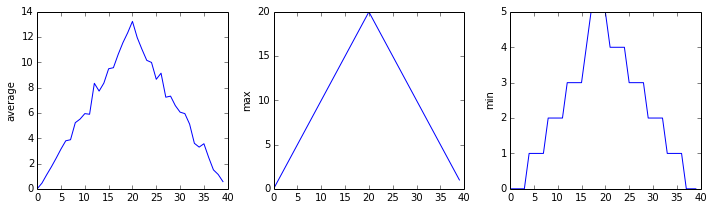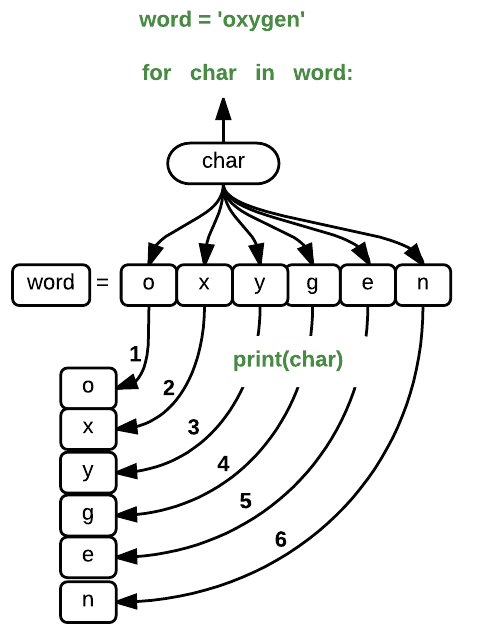# Programming with Python

## Learning Objectives

• Explain what a for loop does.
• Correctly write for loops to repeat simple calculations.
• Trace changes to a loop variable as the loop runs.
• Trace changes to other variables as they are updated by a for loop.

In the last lesson, we wrote some code that plots some values of interest from our first inflammation dataset, and reveals some suspicious features in it, such as from inflammation-01.csvWe have a dozen data sets right now, though, and more on the way. We want to create plots for all of our data sets with a single statement. To do that, we’ll have to teach the computer how to repeat things.

An example task that we might want to repeat is printing each character in a word on a line of its own.

word = 'lead'

We can access a character in a string using its index. For example, we can get the first character of the word ‘lead’, by using word. One way to print each character is to use four print statements:

print(word)
print(word)
print(word)
print(word)
l
e
a
d

This is a bad approach for two reasons:

1. It doesn’t scale: if we want to print the characters in a string that’s hundreds of letters long, we’d be better off just typing them in.

2. It’s fragile: if we give it a longer string, it only prints part of the data, and if we give it a shorter one, it produces an error because we’re asking for characters that don’t exist.

word = 'tin'
print(word)
print(word)
print(word)
print(word)
t
i
n
---------------------------------------------------------------------------
IndexError                                Traceback (most recent call last)
<ipython-input-3-7974b6cdaf14> in <module>()
3 print(word)
4 print(word)
----> 5 print(word)

IndexError: string index out of range

Here’s a better approach:

word = 'lead'
for char in word:
print(char)
l
e
a
d

This is shorter—certainly shorter than something that prints every character in a hundred-letter string—and more robust as well:

word = 'oxygen'
for char in word:
print(char)
o
x
y
g
e
n

The improved version uses a for loop to repeat an operation—in this case, printing—once for each thing in a collection. The general form of a loop is:

for variable in collection:
do things with variable

Using the oxygen example above, the loop might look like this:Where each character (char) in the variable word is looped through and printed one character after another. The numbers in the diagram denote which loop cycle the character was printed in (1 being the first loop, and 6 being the final loop).

We can call the loop variable anything we like, but there must be a colon at the end of the line starting the loop, and we must indent anything we want to run inside the loop. Unlike many other languages, there is no command to signify the end of the loop body (e.g. end for); what is indented after the for statement belongs to the loop.

Here’s another loop that repeatedly updates a variable:

length = 0
for vowel in 'aeiou':
length = length + 1
print('There are', length, 'vowels')
There are 5 vowels

It’s worth tracing the execution of this little program step by step. Since there are five characters in 'aeiou', the statement on line 3 will be executed five times. The first time around, length is zero (the value assigned to it on line 1) and vowel is 'a'. The statement adds 1 to the old value of length, producing 1, and updates length to refer to that new value. The next time around, vowel is 'e' and length is 1, so length is updated to be 2. After three more updates, length is 5; since there is nothing left in 'aeiou' for Python to process, the loop finishes and the print statement on line 4 tells us our final answer.

Note that a loop variable is just a variable that’s being used to record progress in a loop. It still exists after the loop is over, and we can re-use variables previously defined as loop variables as well:

letter = 'z'
for letter in 'abc':
print(letter)
print('after the loop, letter is', letter)
a
b
c
after the loop, letter is c

Note also that finding the length of a string is such a common operation that Python actually has a built-in function to do it called len:

print(len('aeiou'))
5

len is much faster than any function we could write ourselves, and much easier to read than a two-line loop; it will also give us the length of many other things that we haven’t met yet, so we should always use it when we can.

## From 1 to N

Python has a built-in function called range that creates a sequence of numbers. Range can accept 1-3 parameters. If one parameter is input, range creates an array of that length, starting at zero and incrementing by 1. If 2 parameters are input, range starts at the first and ends just before the second, incrementing by one. If range is passed 3 parameters, it starts at the first one, ends just before the second one, and increments by the third one. For example, range(3) produces the numbers 0, 1, 2, while range(2, 5) produces 2, 3, 4, and range(3, 10, 3) produces 3, 6, 9. Using range, write a loop that uses range to print the first 3 natural numbers:

1
2
3

## Computing powers with loops

Exponentiation is built into Python:

print(5 ** 3)
125

Write a loop that calculates the same result as 5 ** 3 using multiplication (and without exponentiation).

## Reverse a string

Write a loop that takes a string, and produces a new string with the characters in reverse order, so 'Newton' becomes 'notweN'.